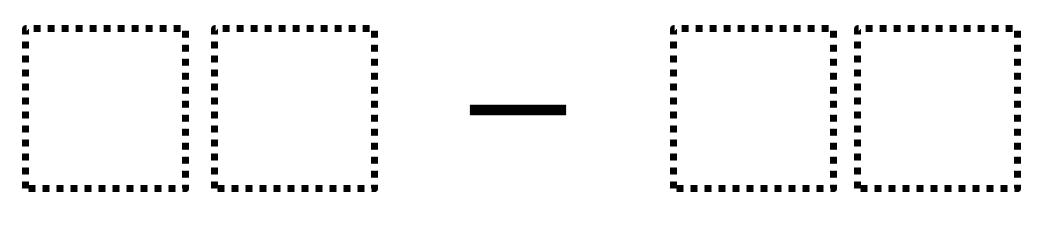Home > Grade 1 > Subtracting Two-Digit Numbers (Elementary)

# Subtracting Two-Digit Numbers (Elementary)

Directions: Using the digits 1 to 9 at most one time each, fill in the boxes to make the smallest (or largest) difference.### Hint

What does the number on the left represent?  What does the number on the right represent?

98 – 12 is the largest difference.  There are several answers for the smallest difference including 81-79.

Source: Robert Kaplinsky

## Sum to 1,000 – Two Addends

Directions: Arrange the digits 1-6 into two 3-digit whole numbers. Make the sum as close …

1.Wouldn’t 20-19 be the smalls difference? or am I missing a given requirement?

Thanks!

•“smallest” I meant. 🙂 Typo!

•Sorry…I see what I did wrong! My mistake!

•Hi Kim. I think it comes down to the reality that “small” and “large” are not mathematically precise words. I have experimented with using phrasing like “least difference” but that seemed to do more harm than good. So, I have stuck with smallest difference and then wind up having a conversation about what is meant by that.

•The problem is a great one! I had just misread it…my mistake. Thanks!

2.I’m doing this now with my 2nd graders and their first question was, “Can we go into the negatives?!” After thinking about this for a moment, I figured that you absolutely could go into the negatives! Is my thinking correct? Here is what they came up with: 12 – 98 = -86 and 97 – 86 = 11. Thanks for stretching our brains!

•Your explorations hit at the heart of the challenge between language that feels comfortable to say out loud and language that is mathematically precise. Specifically, what does “smallest difference” mean?

Is -90 a smaller difference than 1? Perhaps “least difference” would be more precise?

Another way to express what I meant by the problem would be to say that if you put the two whole numbers on a number line, then I want the space between them to be the least (or smallest) possible. Where it gets icky is how -86 < 2 but in this context, is a larger difference. Thoughts?

•Would it be okay to preface the problem with the statement, Two numbers are on a number line, make the largest or smallest difference between them…